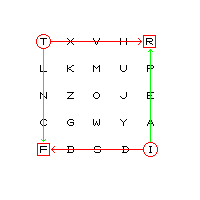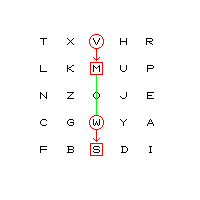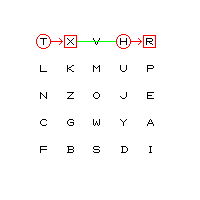[Next] [Up/Previous] [Index]
Main : Index : Pencil and Paper Systems : Improving Substitution : Polygraphic Ciphers and Fractionation : Playfair and its Relatives

# Playfair and its Relatives

Since 26 by 26 tables are awkward and bulky, and certainly impossible to memorize, various systematic methods were developed to encipher more than one letter at a time.

### Playfair

The most famous polygraphic system is, of course, the Playfair cipher, which works as follows: given a 5 by 5 square, containing a jumbled alphabet, such as:

```T X V H R
L K M U P
N Z O J E
C G W Y A
F B S D I
```

doing without one letter by some rule: i.e. if Q is omitted, as here, use KW to stand for QU; or treat I and J, or U and V, as one letter.

Then, a pair of letters is converted to a ciphertext pair using one of three possible rules, whichever one applies:

if the two letters are neither in the same row or the same column, replace each letter by the one that is in its own row, but in the column of the other plaintext letter. Examples: TI becomes RF, TW becomes VC, KA becomes PG, UB becomes KD, WX becomes GV

```T------>R   T-->V H R
| K M U |   | K | U P
| Z O J |   | Z | J E
| G W Y |   C<--W Y A
F<------I   F B S D I
```if the two letters are both in the same column, replace each one by the one below it, wrapping around if necessary. Examples: VW becomes MS, TN becomes LC, TL becomes LN, TF becomes LT, KB becomes ZX

```T X V H R
L K M U P
N Z | J E
C G W Y A
F B S D I
```it the two letters are both in the same row, replace each one by the one to the right of it, wrapping around if necessary. Examples: TH becomes XR, KP becomes ML, NZ becomes ZO.

```T X---H R
L K M U P
N Z O J E
C G W Y A
F B S D I
```Double letters aren't allowed within a single digraph, and must be split up by inserting a letter used as a null (for example, an X) between them.

If the Playfair cipher is used on a computer, perhaps in combination with other ciphers, it might be more convenient to make a rule for double letters, such as using the letter that is both below and to the right of the plaintext letter, and also doubling it. Then, EE would become CC.

### The Four-Square and Two-Square Ciphers

Playfair has inspired some related bigraphic ciphers that, on the one hand, improve security by involving multiple, unrelated alphabets, but on the other hand, are simpler in that they use fewer rules than Playfair.

In the Four-Square cipher, two squares are used to find the two plaintext letters, and two others are used to find the two ciphertext letters:

``` D W X Y M  |  E P T O L     D W X Y M  |  E P T O L
R J E K I  |  C V I Y Z     R J E K I  |  C V I Y Z
U V H P S  |  R M A G B     U V H---------->M A G B
A L B Z N  |  F W J H S     A L | Z N  |  F | J H S
G C O F T  |  U N D X K     G C | F T  |  U | D X K
-----------|-----------     ----|------|----|------
J T B U E  |  V I M A G     J T | U E  |  V | M A G
Z H N D X  |  S W P O H     Z H N<----------W P O H
L A F R G  |  U T Z K E     L A F R G  |  U T Z K E
P M I Y C  |  N R D X Y     P M I Y C  |  N R D X Y
V S K W O  |  B J L C F     V S K W O  |  B J L C F
```

and the only rule for finding the substitute for a digraph is similar to the first rule given for Playfair: the first letter is replaced by the letter in its row, and the second letter's column, and so on. Thus, as the diagram above illustrates, HW becomes MN.

With only two squares, the first plaintext letter and the second ciphertext letter are found in the square on the left, and if both plaintext letters are in the same row, each one is replaced by the other letter. (Other rules are possible, as long as the result is a pair of letters, the first from the square on the right, the second from the square on the left, in the same row. For example, each letter could be replaced by the letter to the right of, or below, the other letter in the other letter's own square.)

During World War II, several digraphic ciphers were used by the German armed forces. All had the common feature that text was broken up by seriation before being enciphered: that is, the message MORE TROOPS NEEDED HERE would be broken up like this:

```MORET NEEDE
ROOPS DHERE
```

and the digraphs to be enciphered would be MR OO RO EP TS ND EH EE DR EE.

While the seriation step is an essential part of some fractionation ciphers we will see below, using it with a digraphic cipher has been questioned, since it allows the cryptanalyst to pretend he is dealing with simple substitution with 26 homophones for each letter, and therefore may make solution easier instead of harder.

According to the book Codebreakers, a collection of reminiscences by those who worked at Bletchley Park during the war, the German army used a cipher in which the digraphs were then enciphered using the two-square cipher. The German Navy used a reciprocal table of digraphs, again after a seriation step, in what was called the Dockyard cipher.

Jim Gillogly has noted that declassified NSA documents refer to another cipher of this type, in which the digraphs were enciphered twice by means of the two-square cipher. Since each letter enciphered the first time was then found in the square on the other side for the second encipherment, the relation between plain and cipher digraphs was much more complicated than in regular Playfair.

[Next] [Up/Previous] [Index]